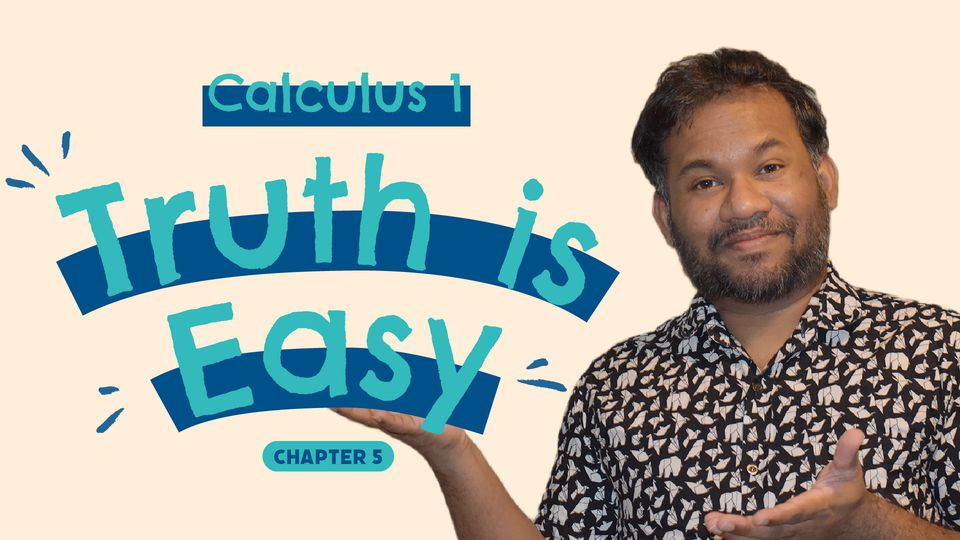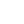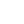Preview the Course
14m

# Calculus 1 - Definite Integral

by Miyuran D. | Math FREE6 Lessons 14:18
1.1. Intro 0:15
2.2. Definition: Definite Integral 2:03
3.3. Continuity Imply Integrability 3:16
4.4. Area as the Limit of a Sum 1:01
5.5. Area as an Integral 0:48
6.6. Properties of Definite Integral 6:55

14m

NGSS Standards

FREE

Course

Here in the section, we introduce what we called a definite integral. If the Riemann sum converges for an integrable function on the closed interval [a,b], then the integration from a to b is called definite integral from a to b. Then we going to see this definite integral give us the area bounded by the curve on a given closed interval. Finally, we talk some of the properties to definite integrals and discuss some questions related to these properties.

In this chapter, we introduce the reversible process of differentiation that is integration. Here we learn rules of integration, definite integrals, and integration techniques.

Calculus is the mathematics of change in motion. Calculus is used to model phenomena that change. Calculus was developed independently in the late 17th century by Isaac Newton and Gottfried Wilhelm Leibniz. Calculus II is all about integral calculus.

Mainly in this course, we are learning a bunch of techniques to solve many different problems with applications. The main topics of calculus we're going to discuss are going to be finding limits: talk about what happens eventually, derivatives: instantaneous rate of change, and integrals: which talk about the area.

This course is with five main chapters:

1 Preliminaries
2. Limit and continuity
3. Differentiation
4. Application of Derivatives
5. Integration

Tags:

• Calculus
• Derivatives
• Functions
• Graphs
• Logarithms
• Math
• STEM

## Instructor

I am Dr. Miuran Dencil, a professional mathematician currently working as an assistant professor. I earned my master’s and doctoral degrees in mathematics in 2016 and 2020 from Texas Tech University, Texas, USA. While in college, I won very prestigious Presidential Graduate Fellowship for 5 years, which is offered to very best graduate student in the school. I was invited speaker for many recognized conferences and won many grants for my research. My dissertation research was Geometric Properties of Special Functions and Related Quadratic Differentials. My research interests include Partial Differential Equations and Number Theory. I graduated from University of Kelaniya, Sri Lanka in 2011 with Bachelor's Degree, which is special degree in mathematics with first class honors.

Teaching is my passion and calling! on top of that mathematics is fun and interactive! I started to teach mathematics at very young age. I have over 16 years of teaching experiences and taught mathematics to kindergarten through senior high school students, college level and graduate level classes.
Once I graduated from my bachelor’s degree, I worked as an instructor at University of Kelaniya from 2011-2013, then worked as a lecturer at university of moratuwa from 2013-2015. Then I started my graduate studies at Texas Tech University while working as GPTI/ Research assistant 2015-2020.
I have taught: Calculus I, II, III + Pre-Cal, AP Cal AB/ AP Cal BC, Trigonometry, Linear Algebra, Geometry, ODE/PDE, Algebra 1,2, Numerical Methods, Real/ Advanced Analysis, Complex Numbers, Discrete Mathematics, Group Theory/ Ring Theory/Number Theory, Integration/ Laplace equations/ Fourier Series- Transformation, Grade Mathematics, Mechanics/ Motion of a particle/ Force- equilibrium/ Harmonic motion, Trainer for exams such as SAT, GRE math sections.

Over 16 years of my teaching experiences and long academic life I have found techniques which helped me to understand and solve any given problem faster and accurate. Now I am ready to teach them to you as I believe nothing is too challenging as long as the student is willing to learn.

As a mathematics is interactive and fun subject it allow students to get extra practice and provide personalized learning atmosphere for students to sharpened their analytical thinking and problem solving technique.

See full profile